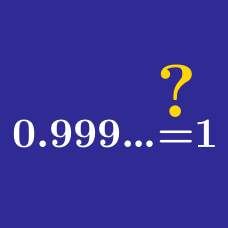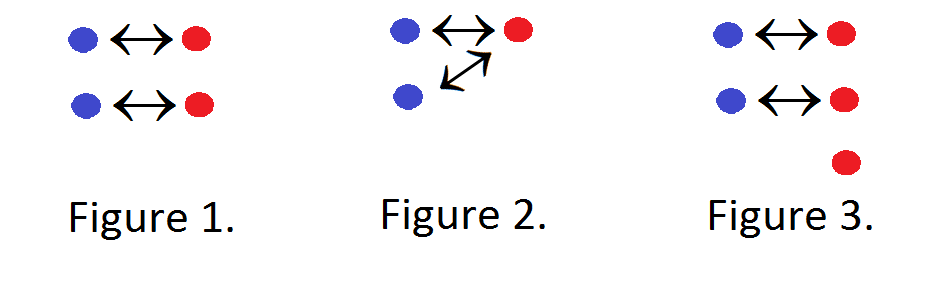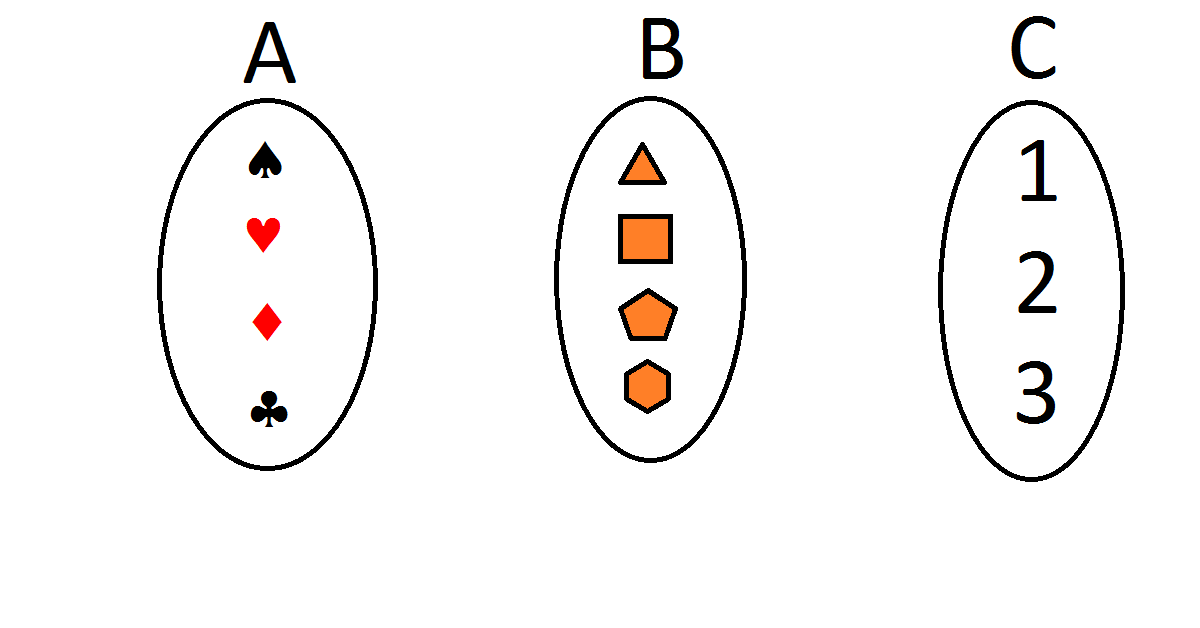Algebra

Infinity MisconceptionsTwo sets have the same cardinality if there is a one-to-one correspondence between all their elements. In the picture above, there is a one-to-one correspondence between the blue dots and the red dots in Figure 1, but there is not a one-to-one correspondence in Figures 2 and 3. Which of the sets below have the same cardinality?Let $A = \{1, 2, 3, 4, 5, \ldots\},$ and $B = \{5, 10, 15, 20, 25, \ldots\}.$ True or False? There is a one-to-one correspondence between the elements of A and the elements of B.

True or False?

There is a one-to-one correspondence between the set $\mathbb{N} = \{0, 1, 2, 3, \ldots \}$ of non-negative and the set $\mathbb{Z} = \{\ldots, -3, -2, -1, 0, 1, 2, 3, \ldots \}$ of integers.

The Hilbert Hotel is a famous hotel with infinitely many rooms: Room 1, Room 2, Room 3, and so on. All the rooms are booked for the night. This time, 100 travelers wander in looking for a place to sleep. The manager wants to figure out a way to accommodate all 100 travelers without moving anyone else out of the hotel. Is this possible?

The Hilbert Hotel is a famous hotel with infinitely many rooms: Room 1, Room 2, Room 3, and so on. All the rooms are booked for the night when infinitely many travelers (Traveler 1, Traveler 2, Traveler 3, ...) wander in, each looking for a room. The manager wants to figure out a way to accomodate all the travelers without moving anyone else out. Is this possible?

×